# IBPS PO Quantitative Aptitude Quiz- 24

IBPS PO Quantitative Aptitude Quiz- 24

Quantitative Aptitude is a very essential and scoring section of almost every competitive exam. To help you all score better in the exams, we are providing you with the IBPS PO Pre Quantitative Aptitude Quiz. In this IBPS PO Pre Quantitative Aptitude Quiz, candidates will be given a full explanation of each question. This IBPS PO Pre Quantitative Aptitude Quiz contains a variety of questions ranging from easy to difficult level. This IBPS PO Pre Quantitative Aptitude Quiz includes all of the most recent pattern-based questions, as well as Previous Year Quantitative Aptitude Questions from banking exams. This IBPS PO Pre Quantitative Aptitude Quiz is available to you at no cost. This IBPS PO Pre Quantitative Aptitude Quiz will assist aspirants in better understanding the topic and learning how to solve tricky problems. Candidates must try to solve this IBPS PO Pre Quantitative Aptitude Quiz to enhance their preparation for upcoming exams.

1. How many 5 letter words can be formed by using letters of “VALAR DOHAERIS” without repetition of letters in which only two vowels comes always.
(a) 14400
(b) 720
(c) 175
(d) 5040
(e) 3600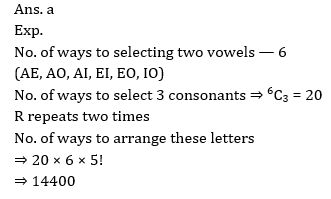2. A sum is invested in a scheme offering C.I. at rate of 20% for first year, 25% for 2nd year and 10% for 3rd year. If difference between 3rd year interest and 2nd year interest is 825 Rs. then find the sum invested.
(a) 5000
(b) 4500
(c) 5500
(d) 3200
(e) 6000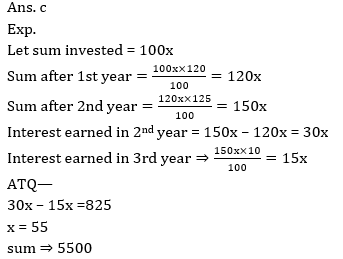3. A, B and C complete a work in 16 days working alternatively starting with A. If efficiency of A is 25% more than the efficiency of B and efficiency of C is 40% less than the efficiency of A then find the time taken by C to complete the whole work alone.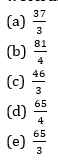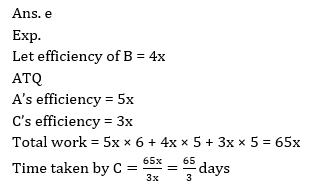4. Find the probability of choosing two even numbers from the set of natural numbers that are co-prime.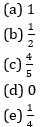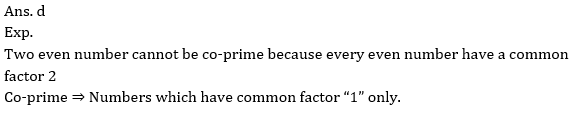5. An article is sold at 10% discount on mark price. If discount is not given then an additional profit of Rs. 96 is earned. If ratio between cost price and mark price is 5 : 6 then find the selling price when article sold at 30% profit.
(a) 1040 Rs.
(b) 960 Rs.
(c) 864 Rs.
(d) 800 Rs.
(e) 1050 Rs.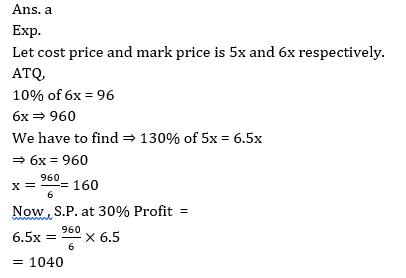Direction (6-10): Find the approximate value that will come in place of question mark(?)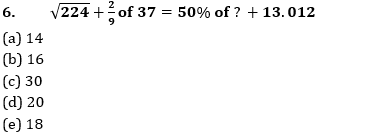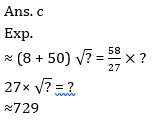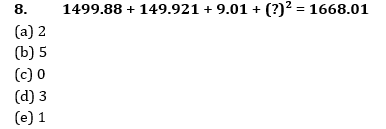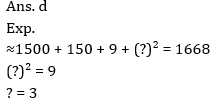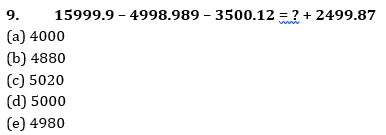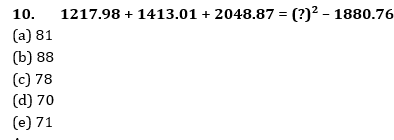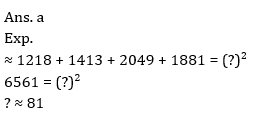###Click to Buy Bank MahaCombo Package

Recommended PDF’s for:

#### Most important PDF’s for Bank, SSC, Railway and Other Government Exam : Download PDF Now

AATMA-NIRBHAR Series- Static GK/Awareness Practice Ebook PDF Get PDF here
The Banking Awareness 500 MCQs E-book| Bilingual (Hindi + English) Get PDF here
AATMA-NIRBHAR Series- Banking Awareness Practice Ebook PDF Get PDF here
Computer Awareness Capsule 2.O Get PDF here
AATMA-NIRBHAR Series Quantitative Aptitude Topic-Wise PDF Get PDF here
AATMA-NIRBHAR Series Reasoning Topic-Wise PDF Get PDF Here
Memory Based Puzzle E-book | 2016-19 Exams Covered Get PDF here
Caselet Data Interpretation 200 Questions Get PDF here
Puzzle & Seating Arrangement E-Book for BANK PO MAINS (Vol-1) Get PDF here
ARITHMETIC DATA INTERPRETATION 2.O E-book Get PDF here
3